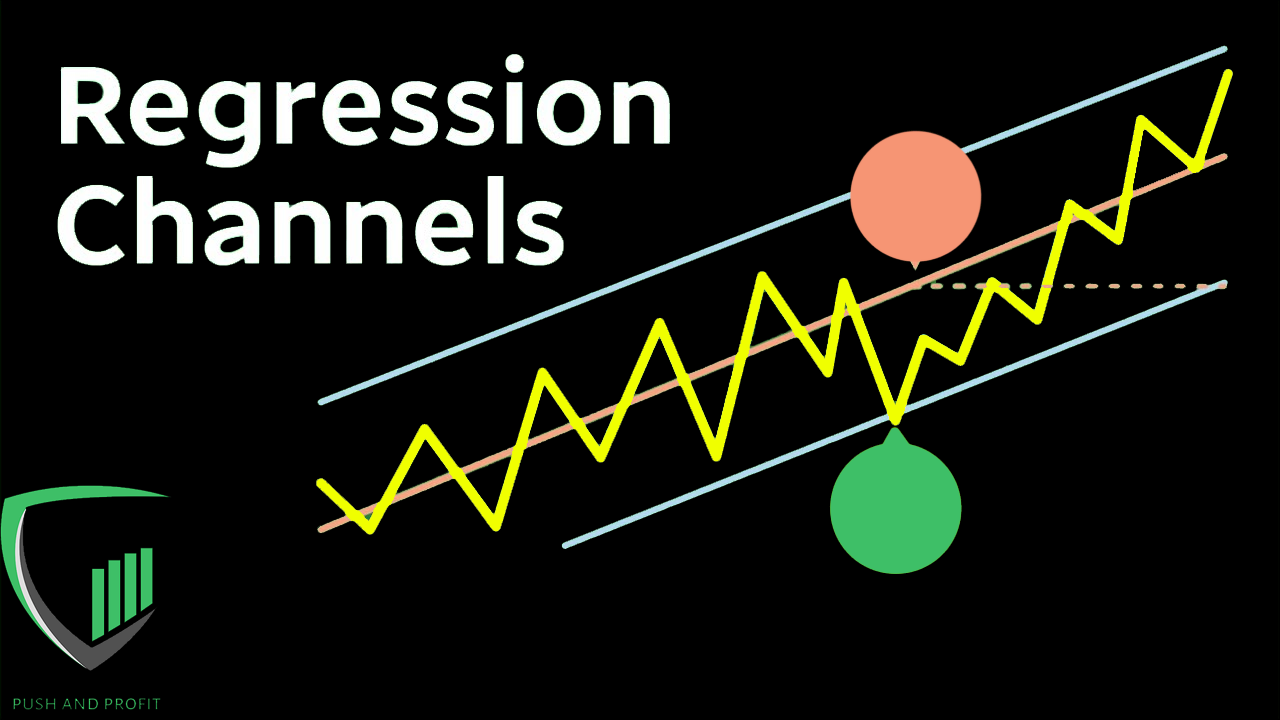# Using Linear Regression Channels To Trade

Apr 23, 2023Linear regression channels are a popular tool used by traders to identify trends in the stock market. This technique helps traders determine whether a stock is trading within a normal range or if it is trending in a particular direction.

• What are Linear Regression Channels?
• How to Calculate Linear Regression Channels
• Using Linear Regression Channels to Make Trading Decisions
• Conclusion

# What are Linear Regression Channels?

Linear regression channels are a type of technical analysis tool that traders use to identify the trend of a stock's price movement. These channels are plotted using a linear regression line, which is a straight line that represents the trend of the stock's price over a period of time.

## How to Calculate Linear Regression Channels

To calculate a linear regression channel, you need to follow these steps:

### Step 1 - Choose a Time Frame

Choose a time frame for your linear regression channel. This can be any period of time that you want to analyze, such as a day, a week, or a month.

### Step 2 - Calculate the Regression Line

Calculate the regression line using the data from the time frame you selected. This is done by finding the slope of the line that best fits the data points.

#### Calculation Formula

The formula for calculating the regression line is: Y = a + bX, where Y is the dependent variable (the stock's price), X is the independent variable (time), a is the intercept of the line, and b is the slope of the line.

### Step 3 - Calculate the Upper and Lower Bounds of the Channel

Calculate the upper and lower bounds of the channel by adding and subtracting a certain percentage of the standard deviation of the regression line from the line itself. This creates a channel that shows the expected range of prices for the stock over the time frame.

#### Upper and Lower Bounds Calculation

• To calculate the upper bound, add a certain percentage of the standard deviation to the regression line.
• To calculate the lower bound, subtract a certain percentage of the standard deviation from the regression line.
• The most commonly used percentage for calculating the bounds is 2.

## Using Linear Regression Channels to Make Trading Decisions

Once you have calculated the linear regression channel, you can use it to make trading decisions. Here are a few ways to use this tool:

If the stock's price is trading within the channel, it indicates that the stock is trading within a normal range. However, if the stock's price breaks above the upper bound of the channel, it is a buy signal. On the other hand, if the stock's price falls below the lower bound of the channel, it is a sell signal.

"When the stock's price breaks above the upper bound of the channel, it is a buy signal. On the other hand, if the stock's price falls below the lower bound of the channel, it is a sell signal."

### Trading Decision 2 - Take Profit or Stop Loss

You can also use the linear regression channel to set profit targets and stop losses. If you have a long position, you may want to take profits when the stock's price reaches the upper bound.